Poker Math: Every Decision is based on Pot Odds

This is a very important lesson and can also be quite intimidating to a lot of people as we are going to discuss Poker Math!

But there is no need for you to be intimidated, Poker Maths is very simple and we will show you a very simple method in this lesson.

You won’t need to carry a calculator around with you or perform any complex mathematical calculations.

What is Poker Math?

As daunting as it sounds, it is simply a tool that we use during the decision making process to calculate the Pot Odds in Poker and the chances of us winning the pot.

Remember, Poker is not based on pure luck, it is a game of probabilities, there are a certain number of cards in the deck and a certain probability that outcomes will occur. So we can use this in our decision making process.

Every time we make a decision in Poker it is a mathematical gamble, what we have to make sure is that we only take the gamble when the odds are on in our favour. As long as we do this, in the long term we will always come out on top.

When to Use Poker Maths

Poker Maths is mainly used when we need to hit a card in order to make our hand into a winning hand, and we have to decide whether it is worth carrying on and chasing that card.

To make this decision we consider two elements:

1. How many “Outs” we have (Cards that will make us a winning hand) and how likely it is that an Out will be dealt.
2. What are our “Pot Odds” – How much money will we win in return for us taking the gamble that our Out will be dealt

We then compare the likelihood of us hitting one of our Outs against the Pot Odds we are getting for our bet and see if mathematically it is a good bet.

The best way to understand and explain this is by using a hand walk through, looking at each element individually first, then we’ll bring it all together in order to make a decision on whether we should call the bet.

Consider the following situation where you hold A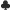8in the big blind. Before the flop everyone folds round to the small blind who calls the extra 5c, to make the Total pot before the Flop 20c (2 players x 10c). The flop comes down K9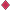4and your opponent bets 10c. Let’s use Poker Math to make the decision on whether to call or not.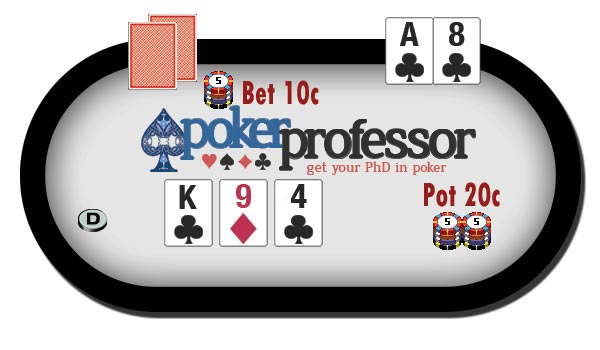Poker Outs

When we are counting the number of “Outs” we have, we are looking at how many cards still remain in the deck that could come on the turn or river which we think will make our hand into the winning hand.

In our example hand you have a flush draw needing only one more Club to make the Nut Flush (highest possible). You also hold an overcard, meaning that if you pair your Ace then you would beat anyone who has already hit a single pair on the flop.

From the looks of that flop we can confidently assume that if you complete your Flush or Pair your Ace then you will hold the leading hand. So how many cards are left in the deck that can turn our hand into the leading hand?

• Flush – There are a total of 13 clubs in the deck, of which we can see 4 clubs already (2 in our hand and 2 on the flop) that means there are a further 9 club cards that we cannot see, so we have 9 Outs here.
• Ace Pair – There are 4 Ace’s in the deck of which we are holding one in our hand, so that leaves a further 3 Aces that we haven’t seen yet, so this creates a further 3 Outs.

So we have 9 outs that will give us a flush and a further 3 outs that will give us Top Pair, so we have a total of 12 outs that we think will give us the winning hand.

So what is the likelihood of one of those 12 outs coming on the Turn or River?

Professor’s Rule of 4 and 2

An easy and quick way to calculate this is by using the Professor’s rule of 4 and 2. This way we can forget about complex calculations and quickly calculate the probability of hitting one of our outs.

The Professor’s Rule of 4 and 2

• After the Flop (2 cards still to come… Turn + River)
Probability we will hit our Outs = Number of Outs x 4
• After the Turn (1 card to come.. River)
Probability we will hit our Outs – Number of Outs x 2

So after the flop we have 12 outs which using the Rule of 4 and 2 we can calculate very quickly that the probability of hitting one of our outs is 12 x 4 = 48%. The exact % actually works out to 46.7%, but the rule of 4 and 2 gives us a close enough answer for the purposes we need it for.

If we don’t hit one of our Outs on the Turn then with only the River left to come the probability that we will hit one of our 12 Outs drops to 12 x 2 = 24% (again the exact % works out at 27.3%)

To compare this to the exact percentages lets take a look at our poker outs chart:

After the Flop (2 Cards to Come) After the Turn (1 Card to Come)
Outs Rule of 4 Exact % Outs Rule of 2 Exact %
1 4 % 4.5 % 1 2 % 2.3 %
2 8 % 8.8 % 2 4 % 4.5 %
3 12 % 13.0 % 3 6 % 6.8 %
4 16 % 17.2 % 4 8 % 9.1 %
5 20 % 21.2 % 5 10 % 11.4 %
6 24 % 25.2 % 6 12 % 13.6 %
7 28 % 29.0 % 7 14 % 15.9 %
8 32 % 32.7 % 8 16 % 18.2 %
9 36 % 36.4 % 9 18 % 20.5 %
10 40 % 39.9 % 10 20 % 22.7 %
11 44 % 43.3 % 11 22 % 25.0 %
12 48 % 46.7 % 12 24 % 27.3 %
13 52 % 49.9 % 13 26 % 29.5 %
14 56 % 53.0 % 14 28 % 31.8 %
15 60 % 56.1 % 15 30 % 34.1 %
16 64 % 59.0 % 16 32 % 36.4 %
17 68 % 61.8 % 17 34 % 38.6 %

As you can see the Rule of 4 and 2 does not give us the exact %, but it is pretty close and a nice quick and easy way to do the math in your head.

Now lets summarise what we have calculated so far:

• We estimate that to win the hand you have 12 Outs
• We have calculated that after the flop with 2 cards still to come there is approximately a 48% chance you will hit one of your outs.

Now we know the Odds of us winning, we need to look at the return we will get for our gamble, or in other words the Pot Odds.

Pot Odds

When we calculate the Pot Odds we are simply looking to see how much money we will win in return for our bet. Again it’s a very simple calculation…

Pot Odds Formula

Pot Odds = Total Pot divided by the Bet I would have to call

What are the pot odds after the flop with our opponent having bet 10c?

• Total Pot = 20c + 10c bet = 30 cents
• Total Bet I would have to make = 10 cents
• Therefore the pot odds are 30 cents divided by 10 cents or 3 to 1.

What does this mean? It means that in order to break even we would need to win once for every 3 times we lose. The amount we would win would be the Total Pot + the bet we make = 30 cents + 10 cents = 40 cents.

Bet number Outcome Stake Winnings
1 LOSE 10 cents Nil
2 LOSE 10 cents Nil
3 LOSE 10 cents Nil
4 WIN 10 cents 40 cents
TOTAL BREAKEVEN 40 cents 40 cents

Break Even Percentage

Now that we have worked out the Pot Odds we need to convert this into a Break Even Percentage so that we can use it to make our decision. Again it’s another simple calculation that you can do in your head.

Break Even Percentage

Break Even Percentage = 100% divided by (Pot odds added together)

Let me explain a bit further. Pot Odds added together means replace the “to” with a plus sign eg: 3 to 1 becomes 3+1 = 4. So in the example above our pot odds are 3 to 1 so our Break Even Percentage = 100% divided by 4 = 25%

Note – This only works if you express your pot odds against a factor of 1 eg: “3 to 1” or “5 to 1” etc. It will not work if you express the pot odds as any other factor eg: 3 to 2 etc.

So… Should You call?

So lets bring the two elements together in our example hand and see how we can use the new poker math techniques you have learned to arrive at a decision of whether to continue in the hand or whether to fold.

To do this we compare the percentage probability that we are going to hit one of our Outs and win the hand, with the Break Even Percentage.

Should I Call?

• Call if……  Probability of Hitting an Out is greater than Pot Odds Break Even Percentage
• Fold if……  Probability of Hitting an Out is less than Pot Odds Break Even Percentage

Our calculations above were as follows:

• Probability of Hitting an Out = 48%
• Break Even Percentage = 25%

If our Probability of hitting an out is higher than the Break Even percentage then this represents a good bet – the odds are in our favour. Why? Because what we are saying above is that we are going to get the winning hand 48% of the time, yet in order to break even we only need to hit the winning hand 25% of the time, so over the long run making this bet will be profitable because we will win the hand more times that we need to in order to just break even.

Hand Walk Through #2

Lets look at another hand example to see poker mathematics in action again.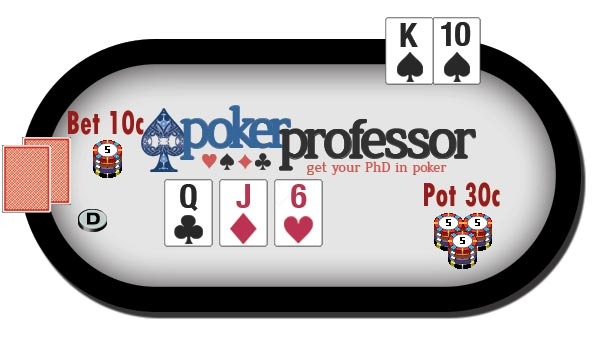Before the Flop:

• Blinds: 5 cents / 10 cents
• Your Position: Big Blind
• Your Hand:  K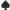10• Before Flop Action: Everyone folds to the dealer who calls and the small blind calls, you check.

Two people have called and per the Starting hand chart you should just check here, so the Total Pot before the flop = 30 cents.

Flop comes down QJ6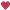and the Dealer bets 10c, the small blind folds.

Do we call? Lets go through the thought process:

How has the Flop helped my hand?
It hasn’t but we do have some draws as we have an open ended straight draw (any Ace or 9 will give us a straight) We also have an overcard with the King.

How has the Flop helped my opponent?
The Dealer did not raise before the flop so it is unlikely he is holding a really strong hand. He may have limped in with high cards or suited connectors. At this stage our best guess is to assume that he has hit top pair and holds a pair of Queens. It’s possible that he hit 2 pair with Q J or he holds a small pair like 6’s and now has a set, but we come to the conclusion that this is unlikely.

How many Outs do we have?
So we conclude that we are facing top pair, in which case we need to hit our straight or a King to make top pair to hold the winning hand.

• Open Ended Straight Draw = 8 Outs (4 Aces and 4 Nines)
• King Top Pair = 3 Outs (4 Kings less the King in our hand)
• Total Outs = 11 Probability of Winning = 11 x 4 = 44%

What are the Pot Odds?
Total Pot is now 40 cents and we are asked to call 10 cents so our Pot odds are 4 to 1 and our break even % = 100% divided by 5 = 20%.

Decision
So now we have quickly run the numbers it is clear that this is a good bet for us (44% vs 20%), and we make the call – Total Pot now equals 50 cents.

Turn Card

Turn Card = 3and our opponent makes a bet of 25 cents.

After the Turn Card
This card has not helped us and it is unlikely that it has helped our opponent, so at this point we still estimate that our opponent is still in the lead with top pair.

Outs
We still need to hit one of our 11 Outs and now with only the River card to come our Probability of Winning has reduced and is now = 11 x 2 = 22%

Pot Odds
The Total Pot is now 75 cents and our Pot odds are 75 divided by 25 = 3 to 1. This makes our Break Even percentage = 100% divided by 4 = 25%

Decision
So now we have the situation where our probability of winning is less than the break even percentage and so at this point we would fold, even though it is a close call.

Summary

Well that was a very heavy lesson, but I hope you can see how Poker Maths doesn’t have to be intimidating, and really they are just some simple calculations that you can do in your head. The numbers never lie, and you can use them to make decisions very easy in Poker.

You’ve learnt some important new skills and it’s time to practise them and get back to the tables with the next stage of the Poker Bankroll Challenge.

Poker Bankroll Challenge: Stage 3

• Stakes: \$0.02/\$0.04
• Buy In: \$3 (75 x BB)
• Starting Bankroll: \$34
• Target: \$9 (3 x Buy In)
• Finishing Bankroll: \$43
• Estimated Sessions: 3

Use this exercise to start to consider your Outs and Pot Odds in your decision making process, and add this tool to the other tools you have already put into practice such as the starting hands chart.